# Pre-Algebra : Additive Inverse Property

## Example Questions

### Example Question #1 : Additive Inverse Property

When is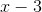neither positive nor negative?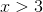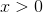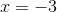Explanation:

The additive inverse ofis. This means that whenis added tothe result is zero.can be written as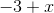.

Since zero is the only number that is neither positive nor negative the answer is.

### Example Question #1 : Additive Inverse Property

What is the additive inverse of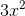?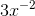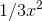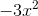Explanation:

The rule for Additive Inverse Property is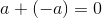.

Using this rule if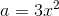,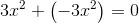So the additive inverse is.

### Example Question #53 : Identities And Properties

Which of the following statements demonstrates the inverse property of addition?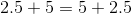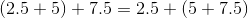None of the examples in the other responses demonstrates the inverse property of addition.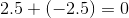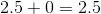Explanation:

The inverse property of addition states that for every real number, a number exists, called the additive inverse, such that the number and its inverse have sum 0. Of the statements given, onlydemonstrates this property, so it is the correct choice.

### Example Question #54 : Identities And Properties

What is the additive inverse of 30?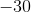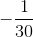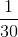Explanation:

The question asks us what number added with 30 will equal zero.  Write the mathematical equation that represents this scenario.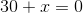Subtract 30 from both sides.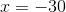### Example Question #55 : Identities And Properties

Which of the following displays the additive inverse property?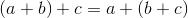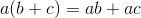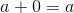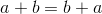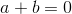Explanation:

The additive inverse property is when two numbers added together equal zero.  So,displays that property.

### All Pre-Algebra Resources